Ms. Espy

•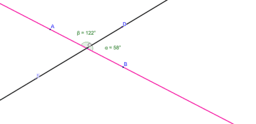Vertical Angle Measures

Activity

Ms. Espy

•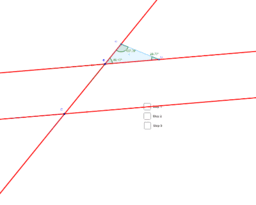Espy Transformations and Parallel Lines

Activity

Ms. Espy

•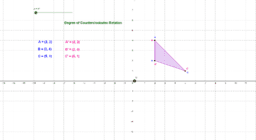Rotations Investigation - Distance and Coordinates

Activity

Ms. Espy

•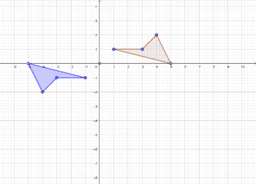1.12 Congruent Polygons

Activity

Ms. Espy

•Volume

Book

Ms. Espy

•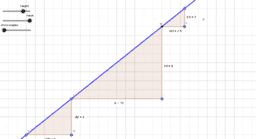Similar Triangles and Slope

Activity

Ms. Espy

•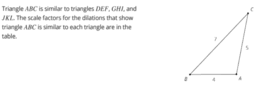Unit 2, Lesson 9: Side Length Quotients in Similar Triangles

Activity

Ms. Espy

•Unit 2, Lesson 4: Dilations on a Square Grid

Activity

Ms. Espy

•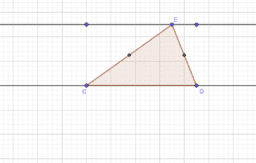Sum of a Triangle's Angles

Activity

Ms. Espy

•Vertical Angle Exploration

Activity

Ms. Espy

•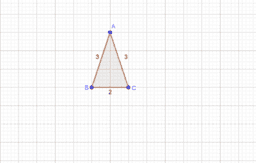1.10 Composing Figures

Activity

Ms. Espy

•Rotation about Point on a Triangle

Activity

Ms. Espy

•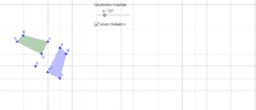Activity

Ms. Espy

•Activity

Ms. Espy

•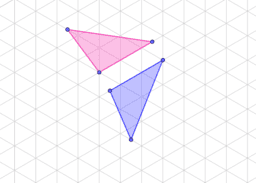Finding the Line of Reflection

Activity

Ms. Espy

•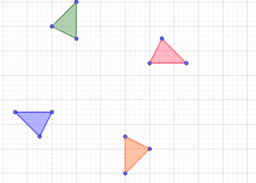Finding the Center of Rotation

Activity

Ms. Espy

•Reflections: Diagonal lines

Activity

Ms. Espy

•Translations Sliders

Activity

Ms. Espy

•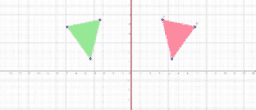Activity

Ms. Espy